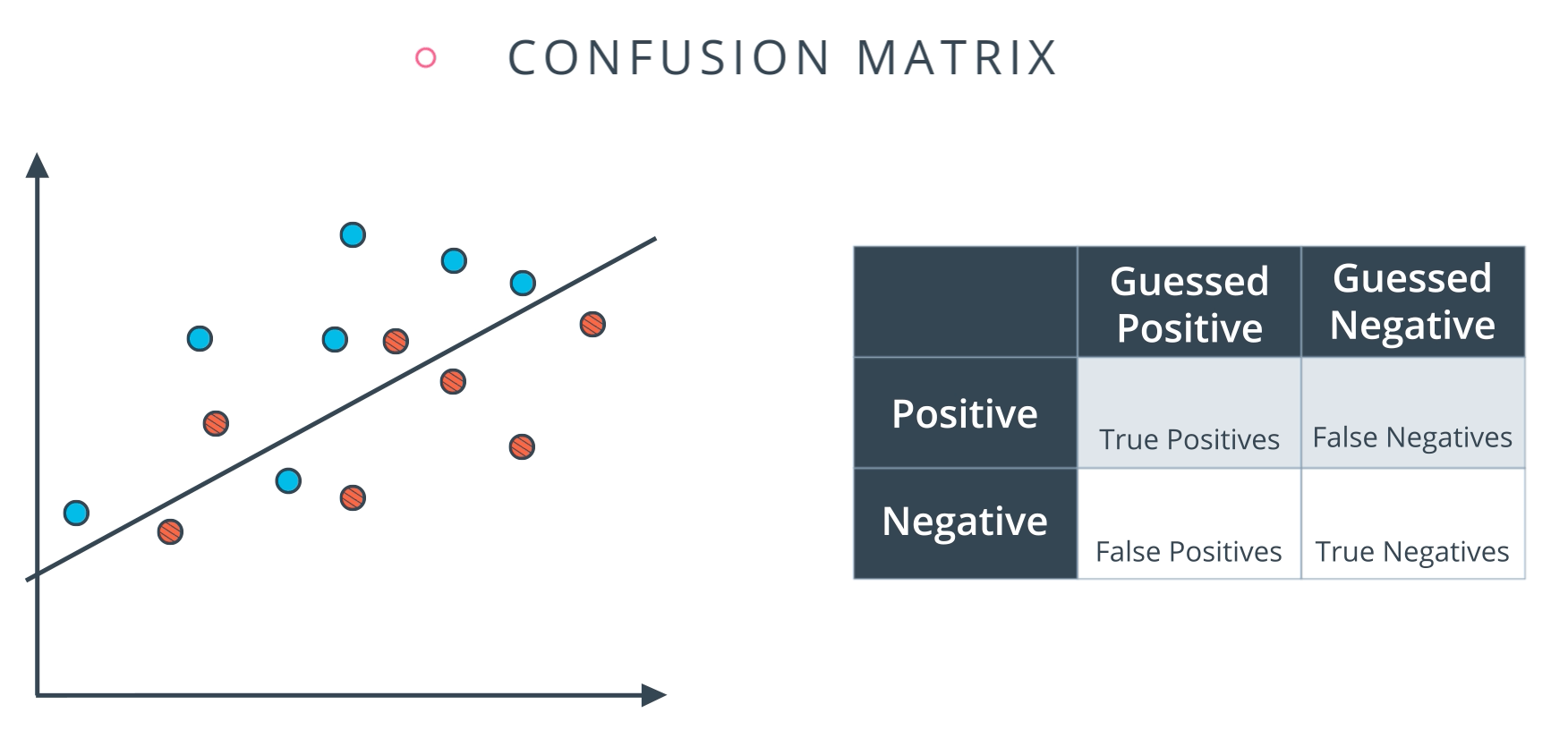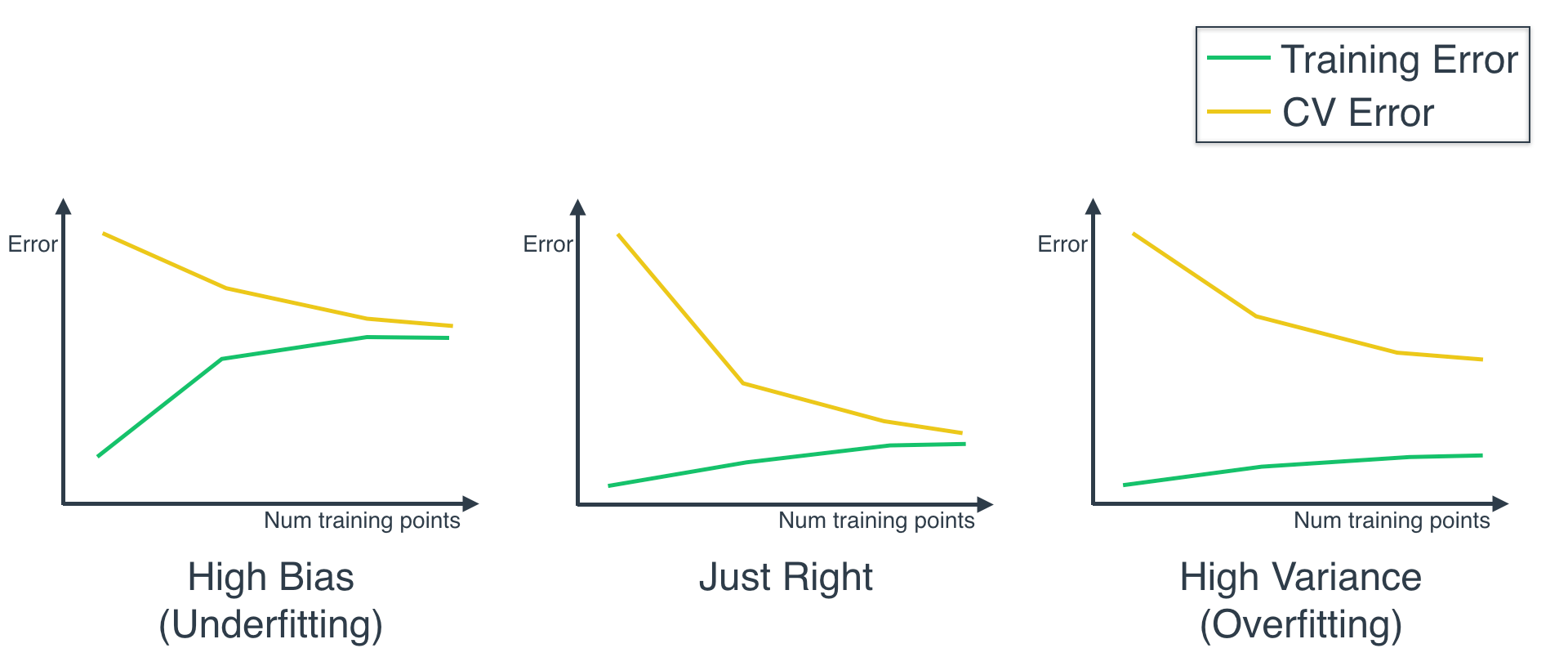Machine learning

Pandas and numpy:

numpy.mean(df[ (df.gold>0) ][“bronze”])

olympic_medal_counts = {‘country_name’:countries,
‘gold’: Series(gold),
‘silver’: Series(silver),
‘bronze’: Series(bronze)}
df = DataFrame(olympic_medal_counts)

del df[‘country_name’]
avg_medal_count=df.apply(lambda x:numpy.mean(x))

b=numpy.array([4,2,1])

a=numpy.column_stack((numpy.array(gold),numpy.array(silver),numpy.array(bronze)))

points=numpy.dot(a,b)

olympic_points_df=DataFrame({‘country_name’:countries,’points’:points})

df[‘points’] = df[[‘gold’,’silver’,’bronze’]].dot([4, 2, 1]) olympic_points_df = df[[‘country_name’,’points’]]

Preprocessing:

For highly-skewed feature distributions such as 'capital-gain' and 'capital-loss', it is common practice to apply a logarithmic transformation on the data so that the very large and very small values do not negatively affect the performance of a learning algorithm. Using a logarithmic transformation significantly reduces the range of values caused by outliers. Care must be taken when applying this transformation however: The logarithm of 0 is undefined, so we must translate the values by a small amount above 0 to apply the the logarithm successfully.

Taking the log of values has the effect of spreading small values and bringing closer to large values.

Scaling:

In addition to performing transformations on features that are highly skewed, it is often good practice to perform some type of scaling on numerical features. Applying a scaling to the data does not change the shape of each feature’s distribution (such as 'capital-gain' or 'capital-loss' above); however, normalization ensures that each feature is treated equally when applying supervised learners. Note that once scaling is applied, observing the data in its raw form will no longer have the same original meaning, as exampled below.

We will use sklearn.preprocessing.MinMaxScaler for this.

If data is not normally distributed, especially if the mean and median vary significantly (indicating a large skew), it is most often appropriate to apply a non-linear scaling — particularly for financial data. One way to achieve this scaling is by using a Box-Cox test, which calculates the best power transformation of the data that reduces skewness. A simpler approach which can work in most cases would be applying the natural logarithm.

Identifying Outliers:

Tukey’s method

from sklearn.model_selection import train_test_split
X_train, X_test, y_train, y_test = train_test_split(X, y, test_size = 0.25)Ifis the mean of the observed data:then the variability of the data set can be measured using three sums of squares formulas:The most general definition of the coefficient of determination isAccuracy = true positive + true negatives/total

from sklearn.metrics import accuracy_score

accuracy_score(y_true,y_pred)

Accuracy is not a right metric when data is skewed.

Precision:Recall:ROC CURVE:

Errors:

Underfitting: Error due to bias, Oversimplified model, performs badly on both training and testing data.

Overfitting: Error due to variance. Over complicated model. model is too specific, performs badly on testing data.

Model complexity graph:Training set: for training model.

Cross validation set: for choosing right parameters like degree of the polynomial. Useful to check whether the trained model is overfitting. If trained model performs poorly on this cross validation set, then the model is overfitted.

Testing set: For final testing.

K-FOLD CROSS VALIDATION:

Divide all your data into k buckets and iteratively create models by choosing one bucket as testing set and remaining for training.

Use average of these models for final model.If you increase training points on different models:Grid Search CV:Plot learning curves to identify when to stop collecting data.

Supervised learning:

If there is an order in output data, then go for continuous model. Ex: income, age.

If there is no order, go for a discrete model. Ex: phone numbers, persons

Algorithms to minimize sum of squared errors:

1. Ordinary least squares: sklearn LinearRegression.

There can be multiple lines that minimize |error|, but only one that minimizes error^2.

Instance based learning:

Properties:

1. Remembers.
2. Fast and doesn’t learn.
3. simple.
4. No generalization.
5. Sensitive to noise.

1. Look up:

In k-nn all features matter equally because when we calculate distance, all features are treated equally.

Locally weighted regression (evolved from k-nearest neighbors):

Naive bayes:

Powerful tools for creating classifiers for incoming labeled data.

Expectation maximization:This is very similar to k-means clustering.

EM is for soft clustering when there is any ambiguity regarding which data point to move to which cluster.

Which supervised classifiers are suitable for numerical as well as categorical data?

The data you have is called ‘mixed data’ because it has both numerical and categorical values. And since you have class labels; therefore, it is a classification problem.  One option is to go with decision trees, which you already tried. Other possibilities are naive Bayes where you model numeric attributes by a Gaussian distribution or so. You can also employ a minimum distance or KNN based approach; however, the cost function must be able to handle data for both types together. If these approaches don’t work then try ensemble techniques. Try bagging with decision trees or else Random Forest that combines bagging and random subspace. With mixed data, choices are limited and you need to be cautious and creative with your choices.

Feature scaling:

To give equal importance to all the different features, we can normalize them to the range of 0 to 1 before applying learning algorithm.

x-x_min/(x_max-x_min)

from sklearn.preprocessing import MinMaxScaler

scaler=MinMaxScaler()

rescaled_weights=scaler.fit_transform(weights)

Feature rescaling would be useful in k-means clustering and rbf svm where we calculate distances but not much in decision trees and linear regression.

Feature selection:

why?

1. Knowledge discovery, interpretability, and insight. To identify which features actually matter among all of them.
2. Curse of dimensionality – The amount of data that you need to train grows exponential to the number of features that you have.

NP-HARD

Feature selection:

Filtering(fast) and Wrapping(slow):

knn suffers from curse of dimensionality because it doesn’t know which features are important. So, we can use decision trees as filtering mechanism to determine important features and then pass them on to knn for learning.

Wrapper methods evaluate subsets of variables which allows, unlike filter approaches, to detect the possible interactions between variables.

Relevance:

1.  A feature is said to be strongly relevant if the Bayes optimal classifier’s performance is strongly affected by the absence of this feature.
2. A feature is weakly relevant if there exists some other feature which can suffice the purpose of this feature.
3. Depends on how much information a feature provides.

Usefulness:

1. Depends on error/model/learner.

Composite feature:When to use PCA?

1. When some latent features are driving the patterns in the data.
2. Dimensionality reduction, reduce noise, better visualization.

Feature transformation:

Independent Component analysis: cocktail party problem.Other feature transformations:

1. Random component analysis – Fast and it usually works.
2. Linear discriminant analysis –

Lesser the cross entropy, better is the model.

cross entropy is the negative logarithm of probabilities of actual events occurring from the perspective of the model we are trying to evaluate.

Difference between RMSE and RMSLE:

RMSLE measures the ratio between actual and predicted.

log(pi+1)log(ai+1)log(pi+1)−log(ai+1)

can be written as log((pi+1)/(ai+1))log((pi+1)/(ai+1))

It can be used when you don’t want to penalize huge differences when both the values are huge numbers.

Also, this can be used when you want to penalize underestimates more than overestimates.

Lets have a look at the below example

Case a) : Pi = 600, Ai = 1000

RMSE = 400, RMSLE = 0.5108

Case b) : Pi = 1400, Ai = 1000

RMSE = 400, RMSLE = 0.3365

As it is evident, the differences are same between actual and predicted in both the cases. RMSE treated them equally however RMSLE penalized the under estimate more than over estimate. Hope this helps.